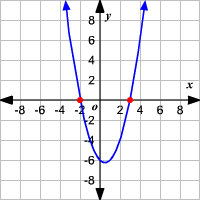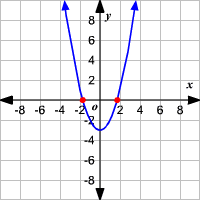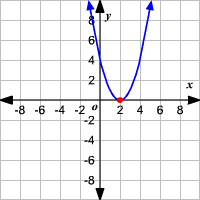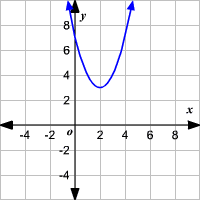# $x$-intercepts of a Quadratic Function

The $x$-intercepts of the function $f\left(x\right)=a{x}^{2}+bx+c,\text{\hspace{0.17em}}\text{\hspace{0.17em}}a\ne 0$ are the solutions of the quadratic equation $a{x}^{2}+bx+c=0$. The solutions of a quadratic equation of the form $a{x}^{2}+bx+c=0$ are given by the quadratic formula.

$x=\frac{-b±\sqrt{{b}^{2}-4ac}}{2a}$

Here, the expression ${b}^{2}-4ac$ is called the discriminant.

The discriminant can be used to confirm the number of $x$-intercepts and the type of solutions of the quadratic equation.

 Value of Discriminant Type and Number of $x$ -intercepts (roots) Example of Graph of Related Function ${b}^{2}-4ac>0;$ ${b}^{2}-4ac$ is a perfect square $2$ real and rational$f\left(x\right)={x}^{2}-x-6$ ${b}^{2}-4ac>0;$ ${b}^{2}-4ac$ is not a perfect square $2$ real and irrational$f\left(x\right)={x}^{2}-3$ ${b}^{2}-4ac=0$ $1$ real$f\left(x\right)={\left(x-2\right)}^{2}$ ${b}^{2}-4ac<0$ No $x$-intercepts$f\left(x\right)={x}^{2}-4x+7$